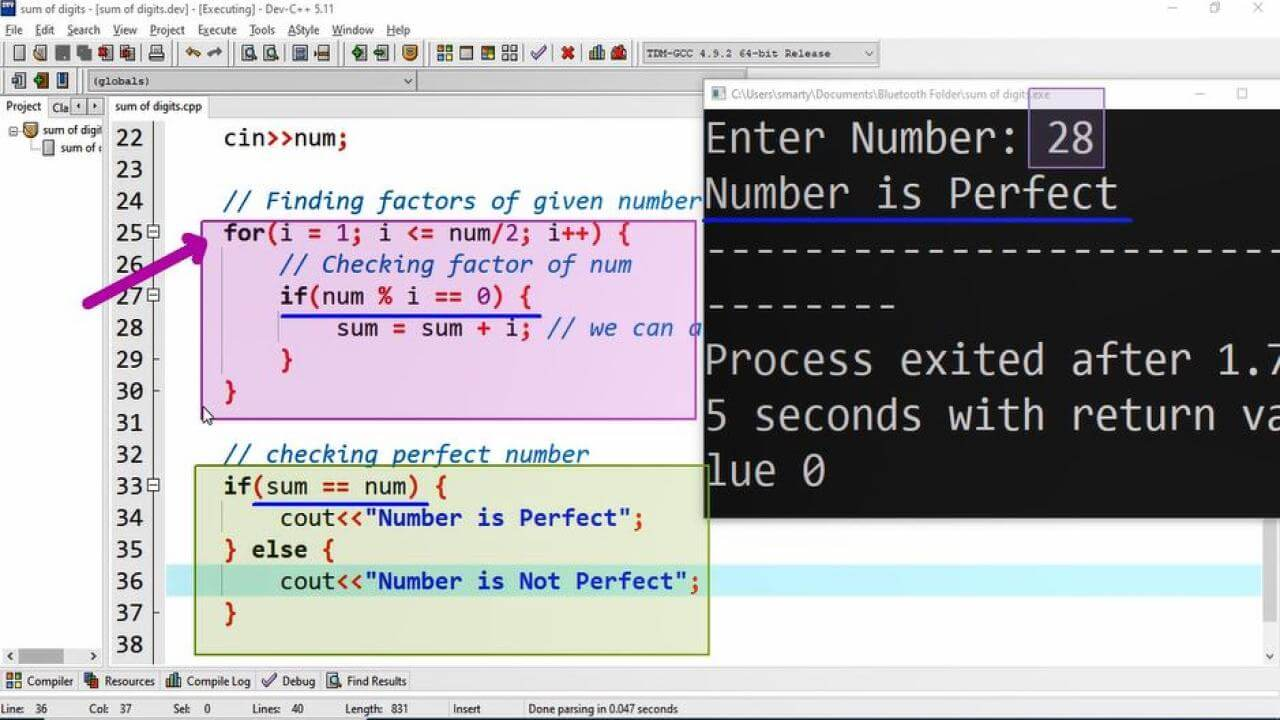# Perfect Number Program Example using CPP

## Source Code:

#include <iostream>

using namespace std;

/*
Number is called Perfect iff
Sum of its factors is equal to number itself
eg:
6 = 1 + 2 + 3 where 1,2,3 are factors of 6. i <= n/2
Some examples of perfect numbers are 6,28,496,8128,.....
*/

int main(int argc, char** argv) {

// Declaration of variables
int num; // used for taking input.
int sum = 0; // used to store sum of factors.
int i; // counter variable

// Taking input in variable num
cout<<"Enter Number: ";
cin>>num;

// Finding factors of given number
for(i = 1; i <= num/2; i++) {
// Checking factor of num
if(num % i == 0) {
sum = sum + i; // we can also use sum++
}
}

// checking perfect number
if(sum == num) { // If true number is perfect
cout<<"Number is Perfect";
} else {
cout<<"Number is Not Perfect";
}

return 0;
}

## Output:## Working:

Every number whose sum of factors is equal to itself is called perfect number. 6 is the smalles perfect number. Furthermore some other perfect numbers are 28, 496, 8128,.......

Let a number N then sum of its factors from 1 to N-1 if equal to N then N is perfect number

In this program example we declare the following variables to implement the logic of program.

 Sr Identifier Type Description 1 num int This variable is used to store user input. 2 sum int sum variable will accumulate factors. 3 i int i is counter variable used in for loop.

After declaration process for loop is used from 1 to N/2, The reason to iterate upto N/2 is that the numbers greater than N/2 will never factors of N. So that we save extra iterations. In every iteration we check either provided counter variable of loop is factor or not using if condition and modulus operator. IF the counter variable is factor then it will add to the sum variable.

Finally we check either sum of factors is equal to provided input number. If this condition is true then it means this number is perfect number otherwise we will print Number is not a perfect number using cout statement.

This program is compiled and tested using Dev-C++ code editor.

## Smallest Perfect Number:

Smallest perfect number is 6. We can also check other perfect numbers using the program discussed above.

## Perfect Number Examples:

Some examples of perfect numbers are 6, 28, 496, 8128,......